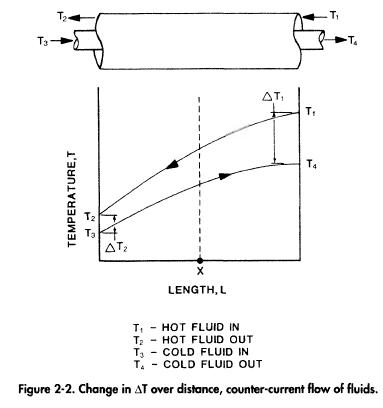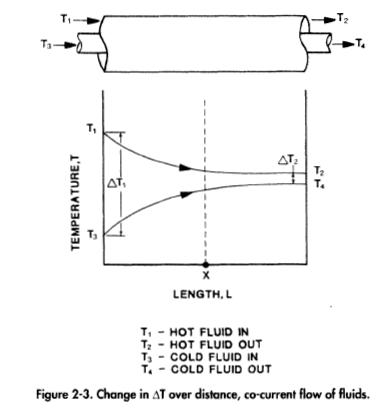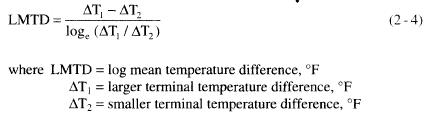# Overall Temperature Difference

The temperature difference may not remain constant throughout the flow path. Plots of temperature vs. pipe length for a system of two concentric pipes in which the annular fluid is cooled and the pipe fluid heated are shown in Figures 2-2 and 2-3. When the two fluids travel in opposite directions, as in Figure 2-2, they are in countercurrent flow. When the fluids travel in the same direction, as in Figure 2-3, they are in co-current flow.The temperature of the inner pipe fluid in either case varies according to one curve as it proceeds along the length of the pipe, and the temperature of the annular fluid varies according to another. The temperature difference at any point is the vertical distance between the two curves.Since the temperature of both fluids changes as they flow through the exchanger, an “average” temperature difference must be used in Equation 2-3. Normally a log mean temperature difference is used and can be found as follows:Although two fluids may transfer heat in either counter-current or cocurrent flow, the relative direction of the two fluids influences the value of the LMTD, and thus, the area required to transfer a given amount ofheat. The following example demonstrates the thermal advantage of using counter-current flow.

Given: A hot fluid enters a concentric pipe at a temperature of 300°F and is to be cooled to 20Q°F by a cold fluid entering at 100°F and heated to 150°F.Equation 2-4 assumes that two fluids are exchanging heat energy while flowing either co-current or counter-current to each other. In many process applications the fluids may flow part of the way in a co-current and the remainder of the way in a counter-current direction. The equations must be modified to model the actual flow arrangement. For preliminary sizing of heat transfer areas required, this correction factor can often be ignored.